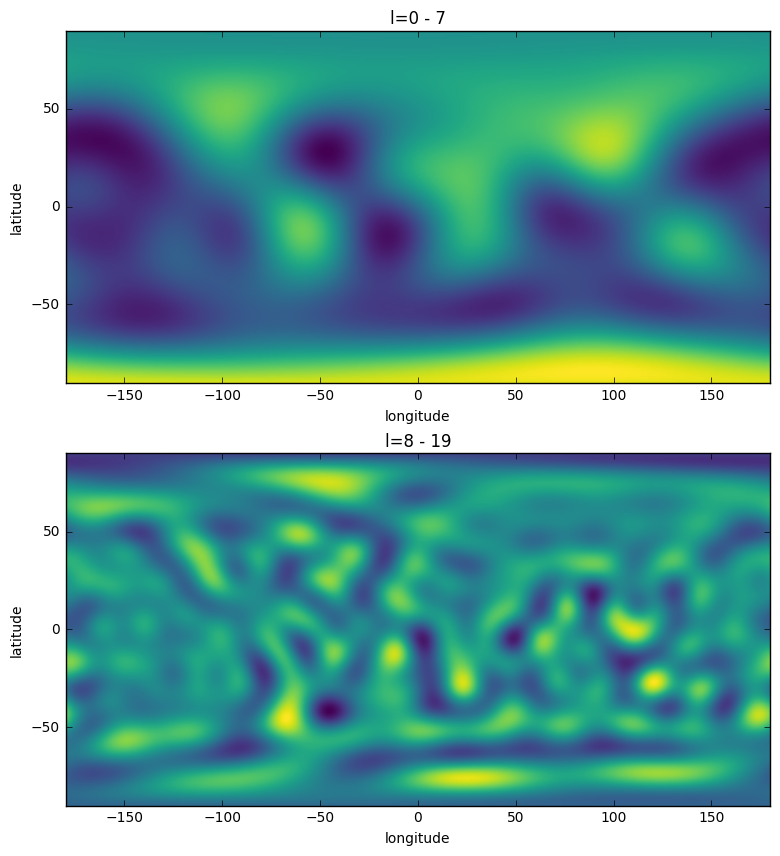# Simple Spherical Harmonic Analyses¶

This tutorial demonstrates how to analyse global data on the sphere using spherical harmonic functions.

In :
%matplotlib inline
from __future__ import print_function # only necessary if using Python 2.x

import matplotlib.pyplot as plt
import numpy as np


In this example, we will use the topography of Earth that is provided in the example directory,

In :
infile = '../ExampleDataFiles/topo.dat.gz'
topo = np.loadtxt(infile)

# plot with matplotlib
fig, ax = plt.subplots(1, 1, figsize=(10, 5))
ax.imshow(topo, extent=(-180, 180, -90, 90), cmap='viridis')
ax.set(xlabel='longitude', ylabel='latitude');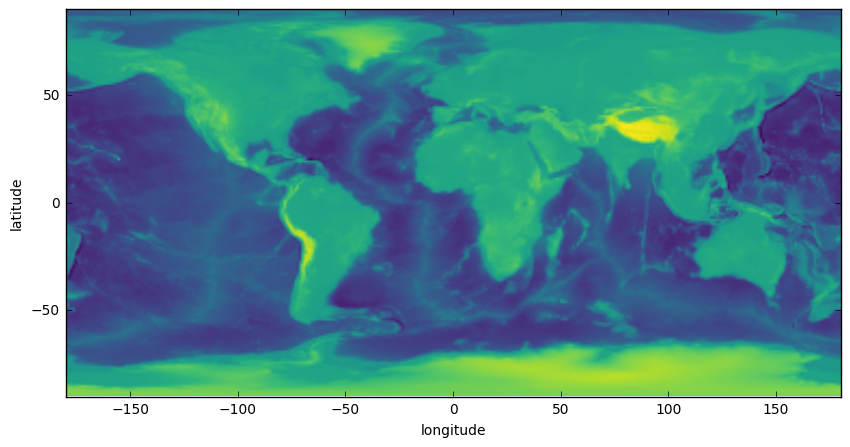Using this data set, we will first demonstrate how to calculate the power spectrum, and second, how to perform simple filtering operations.

## The power spectrum¶

The power spectrum of a function describes how the variance of the function is distributed as a function of spherical harmonic degree. Concentration of power (or energy) in spherical harmonics with a particular wavelength can give hints about the origin of the feature. First, let's import the required functions, and expand the grid into spherical harmonics:

In :
from pyshtools.expand import SHExpandDH;
from pyshtools.spectralanalysis import spectrum

coeffs = SHExpandDH(topo, sampling=2)
nl = coeffs.shape
ls = np.arange(nl)


The "power spectrum" can be calculated using different conventions. The default in pyshtools is to calculate the total power of all angular orders as a function of spherical harmonic degee, which corresponds to the option unit='per_l'. The power per degree l is somewhat equivalent to the power at a given wavenumber magnitude |k| in 2D Fourier analyses.

In :
power_per_l = spectrum(coeffs)

fig, ax = plt.subplots(1, 1, figsize=(10, 5))
ax.plot(ls, power_per_l)
ax.set_yscale('log')
ax.set_xscale('log')
ax.grid()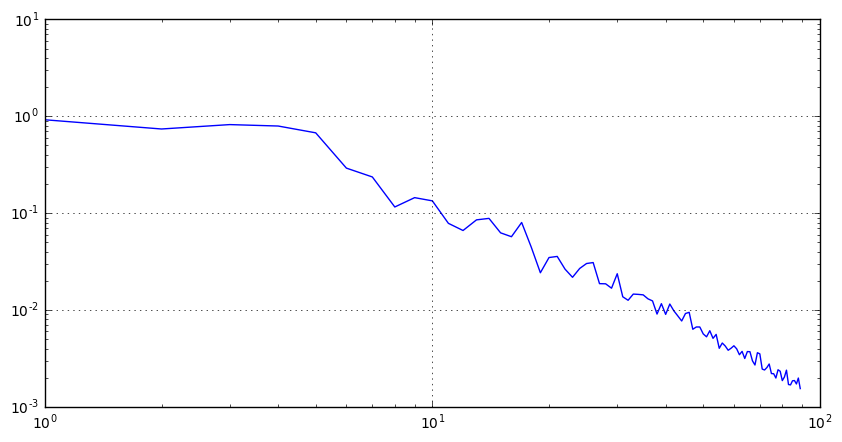The average power per coefficient as a function of spherical harmonic degree can be calculated by setting the parameter unit equal to 'per_lm'. This is simply the power per degree divided by (2l+1), and is analogous to the power per wavenumber kx and ky in 2D Fourier analyses.

In :
power_per_lm = spectrum(coeffs, unit='per_lm')

fig, ax = plt.subplots(1, 1, figsize=(10, 5))
ax.plot(ls, power_per_lm)
ax.set_yscale('log')
ax.set_xscale('log')
ax.grid()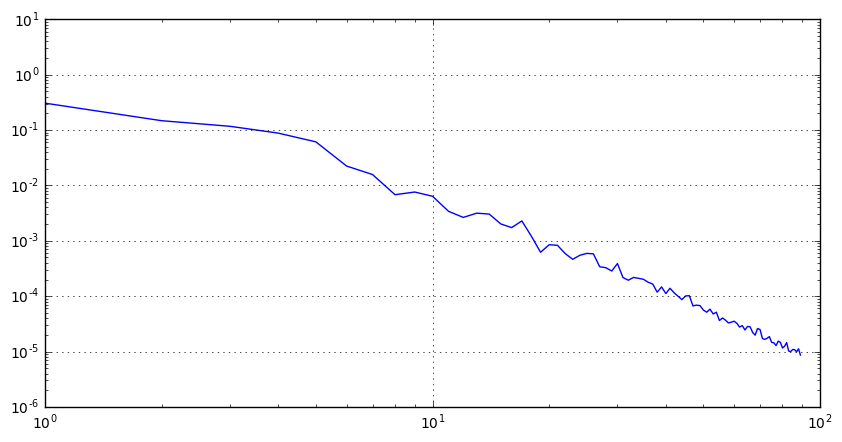Finally, the contribution to the total power from all angular orders over an infinitessimal logarithmic degree band dlog_a(l) can be calculated by setting unit='per_dlogl'. In this case, the contrubution in the band dlog_a(l) is spectrum(l, 'per_dlogl')*dlog_a(l), where a is the base, and where spectrum(l, 'per_dlogl) is equal to spectrum(l, 'per_l')*l*log(a). This power spectrum is useful to analyse dominant scales.

In :
power_per_dlogl = spectrum(coeffs, unit='per_dlogl', base=2.)

fig, ax = plt.subplots(1, 1, figsize=(10, 5))
ax.plot(ls, power_per_dlogl)
ax.set_yscale('log', basey=2)
ax.set_xscale('log', basex=2)
ax.grid()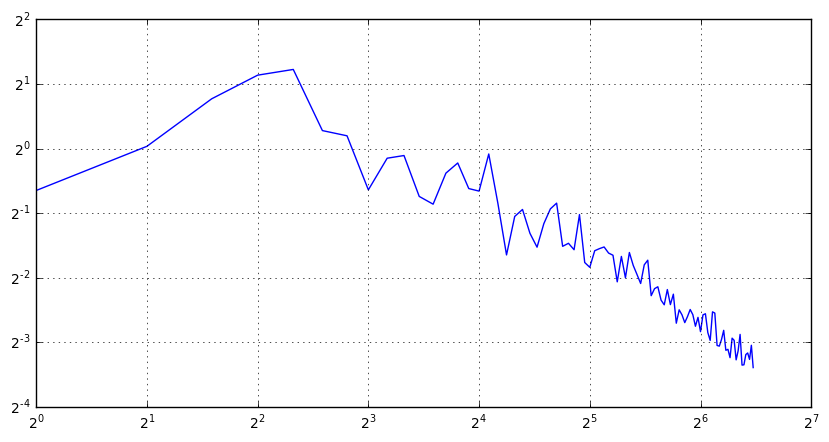## Simple Filtering¶

A global dataset can be filtered isostropically by multiplying the spherical harmonic coefficients by a degree-dependent function. We demonstrate this by setting the coefficients greater or equal to degree 8 equal to zero.

In :
from pyshtools.expand import MakeGridDH;

coeffs_filtered = coeffs.copy()
lmax = 8
coeffs_filtered[:, lmax:, :] = 0.

topo_filtered = MakeGridDH(coeffs_filtered, sampling=2)


Next, we bandpass filter the data to retain only spherical harmonic coefficients between 8 <= l < 20.

In :
coeffs_filtered2 = coeffs.copy()
lmin, lmax = 8, 20
coeffs_filtered2[:, :lmin, :] = 0.
coeffs_filtered2[:, lmax:, :] = 0.

topo_filtered2 = MakeGridDH(coeffs_filtered2, sampling=2)


Finally, let's plot the two filtered data sets:

In :
fig, (row1, row2) = plt.subplots(2, 1, figsize=(10, 10))
row1.imshow(topo_filtered, extent=(-180, 180, -90, 90), cmap='viridis')
row1.set(xlabel='longitude', ylabel='latitude', title='l=0 - 7');
row2.imshow(topo_filtered2, extent=(-180, 180, -90, 90), cmap='viridis')
row2.set(xlabel='longitude', ylabel='latitude', title='l=8 - 19');